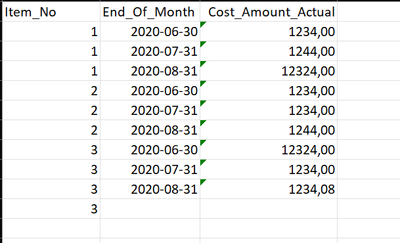cancel
Showing results for
Did you mean:Frequent Visitor

## Create table with group and cummulative sum

Hi all. Could use some help 🙏
Not sure if this is done easier in Power Query or DAX, either works for me.

I need to create a custom table based off of transactions for stock changes. The tricky part is that the correct sum at the end of the month i the total sum of ALL transactions up till that date. I then need to group these per Item and month. Example below:

Here is an extract of the current table and I need to create a new table grouped by Item and whole month with the sum of all previous cost amounts up till that month.The end result would be something like this. Item_No summerized, whole month and sum of cost up till that end of month (note not only sum within that month but sum of all previous transaction for that item)Any help much appreciated

1 ACCEPTED SOLUTIONSuper User

@Hannisky , Create a date table, join with date of you table, add month end date date if needed as column

and try a measure like

Cumm Sales = CALCULATE(SUM(Table[Cost Amount Actual]),filter(all('Date'),'Date'[date] <=max('Date'[date])))

Cumm Sales = CALCULATE(SUM(Table[Cost Amount Actual]),filter(allselected('Date'),'Date'[date] <=max('Date'[date])))Super User

@Hannisky , Create a date table, join with date of you table, add month end date date if needed as column

and try a measure like

Cumm Sales = CALCULATE(SUM(Table[Cost Amount Actual]),filter(all('Date'),'Date'[date] <=max('Date'[date])))

Cumm Sales = CALCULATE(SUM(Table[Cost Amount Actual]),filter(allselected('Date'),'Date'[date] <=max('Date'[date])))Announcements#### Exclusive opportunity for Women!

Join us for a free, hands-on Microsoft workshop led by women trainers for women where you will learn how to build a Dashboard in a Day!#### Power Platform Conference-Power BI and Fabric Sessions

Join us Oct 1 - 6 in Las Vegas for the Microsoft Power Platform Conference.Top Solution Authors
Top Kudoed Authors
Users online (2,339)